In :
import numpy as np
import matplotlib.pyplot as plt
%matplotlib inline

In :
a = np.arange(2*3*4).reshape((2,3,4))
a

Out:
array([[[ 0,  1,  2,  3],
[ 4,  5,  6,  7],
[ 8,  9, 10, 11]],

[[12, 13, 14, 15],
[16, 17, 18, 19],
[20, 21, 22, 23]]])
In :
s = a.itemsize
s

Out:
8
In :
a.dtype

Out:
dtype('int64')

What will be shape of array of windows? In general, it will be a tuple of

• number of images
• number of windows along rows of an image
• number of windows along columns of an image
• number of elements along rows of a window
• number of elements along columns of a window

nWe have 2 images, so first dimension will be 2. If we make 2x2 windows that shift by 1 index horizontally and vertically, we will end of with 2 windows along row dimension and 3 along column dimension. So now we know the shape will start with (2, 2, 3). The last two shape values are the shape of each window, which is 2 x 2. Whole shape will be (2, 2, 3, 2, 3).

Now for the strides. The strides, in genreal will be a tuple of increments need to jump

• from first element of the first window of the first image to the first element of the first window of the second image
• from first element of a window to the first element of the next window below
• from first element of a window to the first element of the next window to the right
• from first element of a row in a window to the first element of the next row in a window
• from an element in a column of a window to the next column

The increment needed to jump from the first element of the first window of the first image, to the first element of the first window of the second image is 12 (the number of values in each image). However, the increment must be in bytes. The number of each element is given by a.itemize. Let's call this $s$. Since each image has 12 elements, the increment will be $12s$. To jump from the first element of a window to the first element of the next window below we will need to jump 4 values because each row of the image has 4 values, or an increment of $4s$. This means we are shifting our windows by one element down each time. We are also shifting our window by one element to the right each time, so we just jump forward only 1 element to the right to start the next window, or $1s$. So far our strides are $(12s, 4s, 1s)$. To jump down or to the right to get the next element in a window, we must stride $4s$ and $1s$. So our strides will be $(12s, 4s, 1s, 4s, 1s)$.

Let's try it.

In :
w = np.lib.stride_tricks.as_strided(a, shape=(2, 2, 3, 2, 2), strides=(12*s, 4*s, 1*s, 4*s, s))

In :
w

Out:
array([[[[[ 0,  1],
[ 4,  5]],

[[ 1,  2],
[ 5,  6]],

[[ 2,  3],
[ 6,  7]]],

[[[ 4,  5],
[ 8,  9]],

[[ 5,  6],
[ 9, 10]],

[[ 6,  7],
[10, 11]]]],

[[[[12, 13],
[16, 17]],

[[13, 14],
[17, 18]],

[[14, 15],
[18, 19]]],

[[[16, 17],
[20, 21]],

[[17, 18],
[21, 22]],

[[18, 19],
[22, 23]]]]])

For each image, we should have 2 x 3 windows, each with 2x2 shape. Let's try indexing into w to get the window second window in the first row of windows from the second image. That should be [[13, 14], [17, 18]] which we can see by staring at a again.

In :
a

Out:
array([[[ 0,  1,  2,  3],
[ 4,  5,  6,  7],
[ 8,  9, 10, 11]],

[[12, 13, 14, 15],
[16, 17, 18, 19],
[20, 21, 22, 23]]])
In :
w[1, 0, 1, :, :]

Out:
array([[13, 14],
[17, 18]])

Okay. Seems to work. Let's try it on an image of a handdrawn digit.

In :
import gzip
import pickle

with gzip.open('mnist.pkl.gz', 'rb') as f:
train_set, valid_set, test_set = pickle.load(f, encoding='latin1')

In :
train_set[:20]

Out:
array([5, 0, 4, 1, 9, 2, 1, 3, 1, 4, 3, 5, 3, 6, 1, 7, 2, 8, 6, 9])
In :
train_set

Out:
7
In :
digit = train_set
digit.shape

Out:
(784,)
In :
digit = digit.reshape((1, 28, 28, 1))

In :
plt.imshow(-digit[0, :, :, 0], interpolation='nearest', cmap='gray')
plt.axis('off');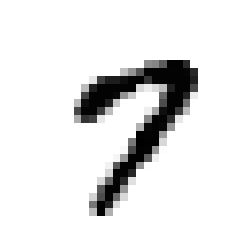Let's try a window shape of 10x10. With an image of 28x28, we will get $28-10+1=19$ windows along rows and also along columns. That's enough to specify the shape of the array containing windows for this one image.

shape=(1, 19, 19, 10, 10)



Now for strides. The first stride is easy. Each image contains 784 values, so it is $784s$. The next two strides, for next window along rows and along columns, will be $28s$ and $1s$. Strides along elements of each window will be the same.

strides=(784*s, 28*s, 1*s, 28*s, 1*s)
In :
s = digit.itemsize
Xw = np.lib.stride_tricks.as_strided(digit, shape=(1, 19, 19, 10, 10), strides=(784*s, 28*s, 1*s, 28*s, 1*s))

In :
Xw.shape

Out:
(1, 19, 19, 10, 10)

Let's try to draw these image patches, arranged in rows and columns corresponding to where they were extracted from the image.

In :
plt.figure(figsize=(12, 12))
ploti = 0
for row in range(19):
for col in range(19):
ploti += 1
plt.subplot(19, 19, ploti)
plt.imshow(-Xw[0, row, col, :, :], interpolation='nearest', cmap='gray')
plt.axis('off')
plt.tight_layout()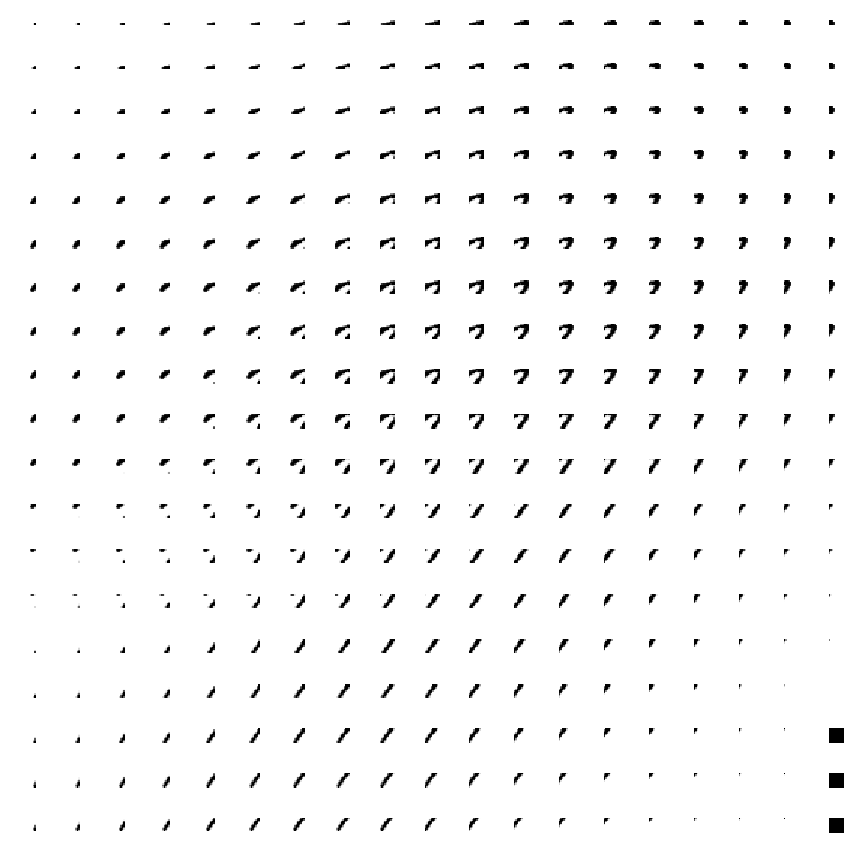Those black squared are being caused by passing to imshow an image with constant values. Let's fix this be specifying a fixed range of image values for all plots. plt.imshow? shows that arguments vmin and vmax can be used for this.

What range should be use?

In :
np.min(-digit), np.max(-digit)

Out:
(-0.99609375, -0.0)
In :
plt.figure(figsize=(12, 12))
ploti = 0
for row in range(19):
for col in range(19):
ploti += 1
plt.subplot(19, 19, ploti)
plt.imshow(-Xw[0, row, col, :, :], interpolation='nearest', cmap='gray', vmin=-1, vmax=0)
plt.axis('off')
plt.tight_layout()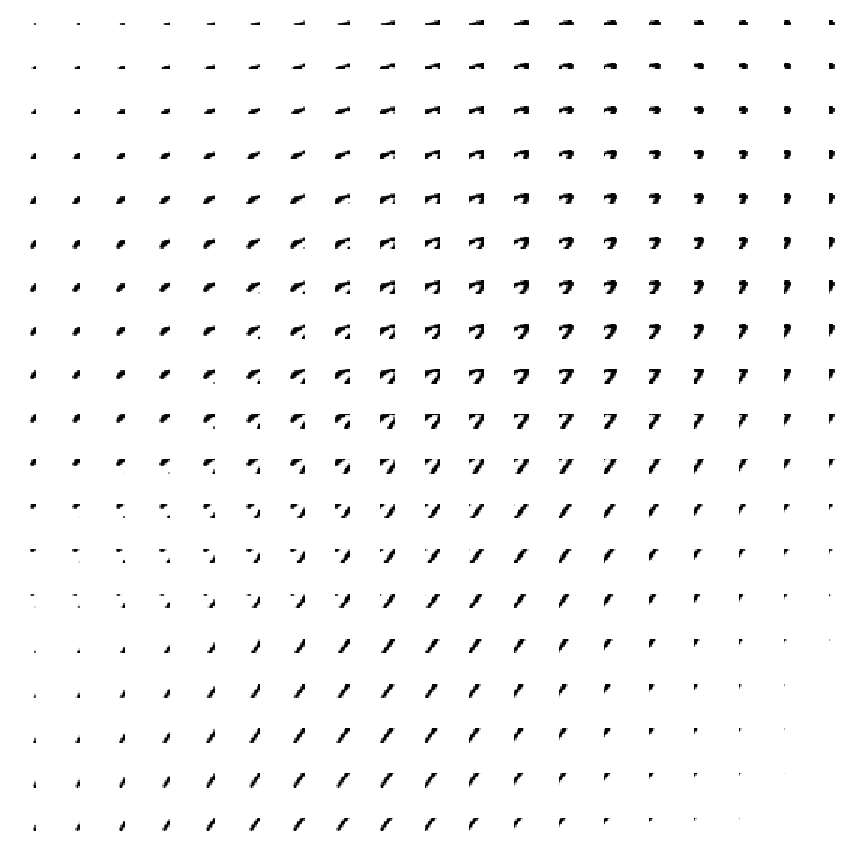By vertically stacking each of these patches, after flattening each into a 10x10 = 100 element vector, they become 19x19 = 361 vectors that make up our windowed representation of this digit.

Passing each of these through a unit having 100 weights (+1 for the bias weight) we have effectively convolved that unit over this digit image. The result is 361 outputs representing a 19x19 grid of values resulting from applying this unit to each 10x10 patch of the image.

For example, let's make a unit that detects a diagonal line from lower left to upper right.

In :
list(range(9,-1,-1))

Out:
[9, 8, 7, 6, 5, 4, 3, 2, 1, 0]
In :
W = np.zeros((10,10))
W[range(9, -1, -1), range(10)] = 1
W *= 0.05
W

Out:
array([[0.  , 0.  , 0.  , 0.  , 0.  , 0.  , 0.  , 0.  , 0.  , 0.05],
[0.  , 0.  , 0.  , 0.  , 0.  , 0.  , 0.  , 0.  , 0.05, 0.  ],
[0.  , 0.  , 0.  , 0.  , 0.  , 0.  , 0.  , 0.05, 0.  , 0.  ],
[0.  , 0.  , 0.  , 0.  , 0.  , 0.  , 0.05, 0.  , 0.  , 0.  ],
[0.  , 0.  , 0.  , 0.  , 0.  , 0.05, 0.  , 0.  , 0.  , 0.  ],
[0.  , 0.  , 0.  , 0.  , 0.05, 0.  , 0.  , 0.  , 0.  , 0.  ],
[0.  , 0.  , 0.  , 0.05, 0.  , 0.  , 0.  , 0.  , 0.  , 0.  ],
[0.  , 0.  , 0.05, 0.  , 0.  , 0.  , 0.  , 0.  , 0.  , 0.  ],
[0.  , 0.05, 0.  , 0.  , 0.  , 0.  , 0.  , 0.  , 0.  , 0.  ],
[0.05, 0.  , 0.  , 0.  , 0.  , 0.  , 0.  , 0.  , 0.  , 0.  ]])
In :
Xw.shape

Out:
(1, 19, 19, 10, 10)
In :
Y = np.tanh(Xw.reshape((19*19, 10*10)) @ W.reshape((-1,1)))

In :
Y.shape

Out:
(361, 1)
In :
Yw = Y.reshape((19,19))
Yw.shape

Out:
(19, 19)
In :
np.min(Yw), np.max(Yw)

Out:
(0.0, 0.4244643684778938)
In :
plt.imshow(-Yw, interpolation='nearest', cmap='gray')

Out:
<matplotlib.image.AxesImage at 0x7ff029c00fd0>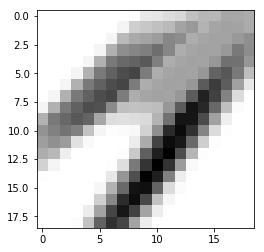To see which parts of the digit image this unit has the highest output, let's draw the patches of the image again with an intensity that is scaled by the unit's output.

In :
plt.figure(figsize=(12, 12))
ploti = 0
for row in range(19):
for col in range(19):
ploti += 1
plt.subplot(19, 19, ploti)
plt.imshow(   -Xw[0,row, col, :, :] * Yw[row, col],   interpolation='nearest', cmap='gray', vmin=-1, vmax=0)
plt.axis('off')
plt.tight_layout()Download conv.tar and extract neuralnetworkConvolutionalClassifier.py, mlutilities.py, and mnist.pkl.gz.

In :
import neuralnetworkConvolutionalClassifier as nnconv
import mlutilities as ml

In :
Xtrain = train_set
Ttrain = train_set.reshape((-1, 1))
Xtest = test_set # .reshape((-1, 28, 28, 1))
Ttest = test_set.reshape((-1, 1))

In [ ]:
retrain = True

if retrain:
nnet = nnconv.NeuralNetworkConvolutionalClassifier(1, [20, 50], 10,
inputShape=(28, 28), windowShape=(5, 5), windowStrides=(4, 4))
nnet.train(Xtrain, Ttrain, 100, verbose=True)
print(nnet)
nnet.save('nnetconvdigits.pkl')
else:
nnet = nnconv.NeuralNetworkConvolutionalClassifier.load('nnetconvdigits.pkl')
print(nnet)

In :
9799 / 60 / 60, 'hours'

Out:
(2.7219444444444445, 'hours')
In :
plt.plot(np.exp(-nnet.getErrors()));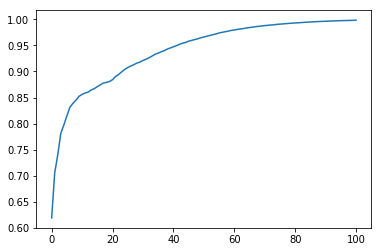In :
Ytrain = nnet.use(Xtrain)
Ytest = nnet.use(Xtest)

In :
def percCorr(a, b):
return np.mean(a == b) * 100

In :
print('\nTraining data: Percent Correct  {:.2f}'.format(percCorr(Ttrain, Ytrain)))
ml.confusionMatrix(Ttrain, Ytrain, range(10))

print('\nTesting data: Percent Correct  {:.2f}'.format(percCorr(Ttest, Ytest)))
ml.confusionMatrix(Ttest, Ytest, range(10));

Training data: Percent Correct  100.00
0    1    2    3    4    5    6    7    8    9
------------------------------------------------------------
0 |100.0  0    0    0    0    0    0    0    0    0
1 |  0  100.0  0    0    0    0    0    0    0    0
2 |  0    0  100.0  0    0    0    0    0    0    0
3 |  0    0    0  100.0  0    0    0    0    0    0
4 |  0    0    0    0  100.0  0    0    0    0    0
5 |  0    0    0    0    0  100.0  0    0    0    0
6 |  0    0    0    0    0    0  100.0  0    0    0
7 |  0    0    0    0    0    0    0  100.0  0    0
8 |  0    0    0    0    0    0    0    0  100.0  0
9 |  0    0    0    0    0    0    0    0    0  100.0

Testing data: Percent Correct  98.24
0    1    2    3    4    5    6    7    8    9
------------------------------------------------------------
0 | 99.2  0    0.2  0    0    0.2  0.2  0.1  0.1  0
1 |  0   99.4  0.3  0    0    0.1  0.1  0.1  0.1  0
2 |  0.2  0.3 98.2  0.3  0    0.1  0    0.5  0.4  0.1
3 |  0    0    0.6 98.3  0    0.7  0    0.2  0.1  0.1
4 |  0    0    0.2  0.1 97.7  0    0.5  0.1  0.1  1.3
5 |  0.1  0    0.2  0.4  0.1 98.3  0.2  0    0.2  0.3
6 |  0.4  0.2  0.3  0.1  0.1  0.2 98.6  0    0    0
7 |  0    0.1  0.9  0.4  0.2  0    0   98.2  0.1  0.2
8 |  0.5  0    0.2  0.2  0.3  0.3  0    0.6 97.5  0.3
9 |  0.3  0.4  0.1  0.2  0.6  0.5  0    0.7  0.3 96.9


Let's see what the first layer of units have learned. An image of the 100 weights in one of those units, reshaped to a 10x10 image patch, might be revealing.

In :
nnet

Out:
NeuralNetworkConvolutionalClassifier(1, [20, 50], 10, (28, 28), (5, 5), (4, 4))
Network was trained for 101 iterations that took 9799.2786 seconds. Final error is 0.002036432373258122.
In :
len(nnet.Vs)

Out:
2
In :
filters = nnet.Vs
filters.shape

Out:
(26, 20)

This makes sense. This layer has 20 units. The weights for one unit are in one column of this weight matrix. Each unit has 101 inputs the 10x10 pixel values in a patch, and one more weight for the bias weight.

In :
plt.figure(figsize=(5, 5))
for u in range(20):
plt.subplot(5, 5, u+1)
plt.imshow(filters[1:,u].reshape((5, 5)), interpolation='nearest', cmap='gray')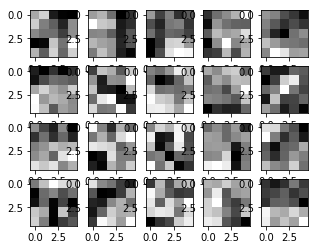In :
classes, Y, Z = nnet.use(Xtest, allOutputs=True)

In :
len(Z)

Out:
2
In :
Z.shape

Out:
(10000, 11520)
In :
Ttest[:5]

Out:
array([,
,
,
,
])
In :
digit = 4

In :
convOutForDigit = Z[digit,:]
convOutForDigit.shape

Out:
(11520,)
In :
11520/20

Out:
576.0
In :
np.sqrt(576)

Out:
24.0
In :
convOutForDigit = convOutForDigit.reshape((24, 24, 20))
convOutForDigit.shape

Out:
(24, 24, 20)
In :
plt.imshow(-Xtest[digit,:].reshape((28, 28)), interpolation='nearest', cmap='gray')

Out:
<matplotlib.image.AxesImage at 0x7f61d6ccc8d0>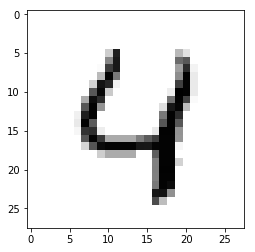In :
plt.figure(figsize=(8, 20))
p = 0
for u in range(20):
p += 1
plt.subplot(20, 2, p)
plt.imshow(filters[1:,u].reshape((5, 5)), interpolation='nearest', cmap='gray')
plt.axis('off')
p += 1
plt.subplot(20, 2, p)
plt.imshow(convOutForDigit[:, :, u], interpolation='nearest', cmap='gray')
plt.axis('off')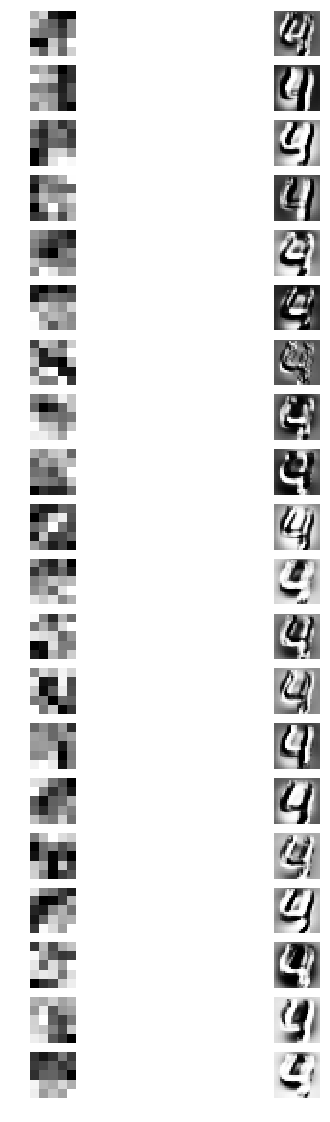In [ ]:


In [ ]: